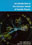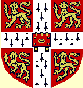© CAMBRIDGE UNIVERSITY PRESS 1998

1.7 Quark Color

Much informative quark physics has been revealed in experiments with e+e- colliding beams. We mention here experiments in the range between centre of mass energies 10 GeV and the threshold energy, around 90 GeV, at which the Z boson can be produced.Figure 1.6. The lowest order Feynman diagram (Chapter 8) for electromagnetic µ+µ- pair production in e+e- collisions.

The e+e- annihilation cross-section(e+e- -> µ+µ-) is comparatively easy to measure, and is easy to calculate in the Weinberg-Salam electroweak theory, which we shall introduce in Chapter 12. At centre of mass energies much below 90 GeV the cross-section is dominated by the electromagnetic process represented by the Feynman diagram of Fig. 1.6. The muon pair are produced ``back-to-back'' in the centre of mass system, which for an e+e- collider is the laboratory system. To leading order in the fine-structure constant= e2 / (40c), the differential cross-section for producing muons moving at an anglewith respect to unpolarised incident beams is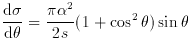(1.1)

where s is the square of the centre of mass energy (see Okun, 1982, p. 205). In the derivation of (1.1) the lepton masses are neglected. Integrating with respect to, the total cross-section is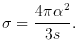(1.2)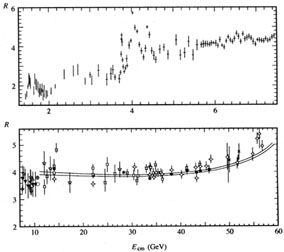Figure 1.7. Measurements of R (E) from the resonance region 1 GeV < E < 11 GeV into the region 11 GeV < E < 60 GeV which contains no prominent resonances and no quark-antiquark production threshold. For E > 11 GeV two curves are shown of calculations that take account of quark colour and include electroweak corrections and strong interaction (QCD) effects. (Adapted from Particle Data Group (1996).)

The quantity R (E) shown in Fig. 1.7 is the ratio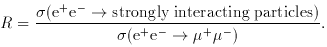(1.3)

At the lower energies many hadronic states are revealed as resonances, but R seems to become approximately constant, R4, at energies above 10 GeV up to about 40 GeV.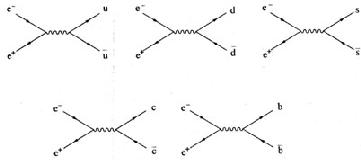Figure 1.8. The lowest order Feynman diagrams for quark-antiquark pair production in e+e- collisions at energies below the Z threshold.

As fundamental particles, quarks have the same electrodynamics as muons, apart from the magnitude of their electric charge. The Feynman diagrams which dominate the numerator of R in this range 10 GeV to 40 GeV are shown in Fig. 1.8. (The top quark has a mass ~ 180 GeV and will not contribute.) For each quark process the formula (1.2) holds, except that e is replaced by the quark's electric charge at the quark vertex, which suggests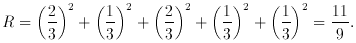(1.4)

This value is too low, by a factor of about 3.

In the Standard Model, the discrepancy is resolved by introducing the idea of quark colour. A quark not only has a flavour index, u, d, s, c, b, t, but also, for each flavour, a colour index. There are postulated to be three basic states of colour, say red, green and blue (r, g, b). With three quark colour states to each flavour, we have to multiply the R of(1.4) by 3, to obtain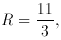(1.5)

which is in excellent agreement with the data of Fig. 1.7.

This invention of colour not only solves the problem of R but, most significantly, solves the problem of the symmetry of the baryon states. We have seen (Section 1.5) that in the absence of any new quantum number baryon states are completely symmetric in the interchange of two quarks. However, if these state functions are multiplied by an antisymmetric colour state function, the overall state becomes antisymmetric, and the Pauli principle is preserved.

Strong support for the mechanism of quark production represented by the Feynman diagrams of Fig. (1.8) is given by other features in the data from e+e- colliders. An e+e- annihilation at high energies produces many hadrons. These are mostly correlated into two back-to-back jets. An example is shown in Fig. 1.9. (The charged particle tracks are curved because of the presence of an external magnetic field: the curvature is related to the particle's momentum.) The direction of a jet may be defined as the direction at the point of production of the total momentum of all the hadrons associated with it. The momenta of two back-to-back jets are equal and opposite. The jet directions may be presumed to be the directions of the initial quark-antiquark pair. This interpretation is corroborated by an examination of the angular distribution of the jet directions of two-jet events from many annihilations, with respect to the e+e- beams. The angular distribution is the same as that for muons (equation (1.1)) after allowance has been made for the Z contribution, which becomes significant as the energy for Z production is approached.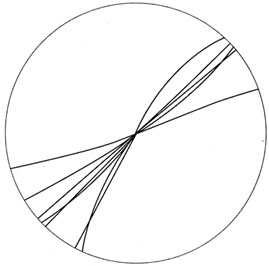Figure 1.9. An example of an e+e- annihilation event that results in two jets of hadrons. The figure shows the projection of the charged particle tracks onto a plan perpendicular to the axis of the e+e- beams. This figure was taken from an event in the TASSO detector at PETRA, DESY.

The hadron jets result from the original quark and antiquark combining with quark-antiquark pairs generated from the vacuum. The precise details of the processes involved are not yet fully understood.